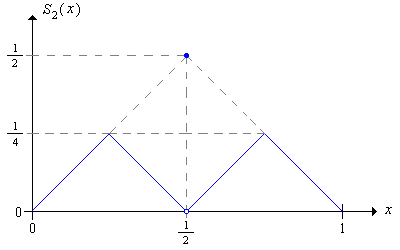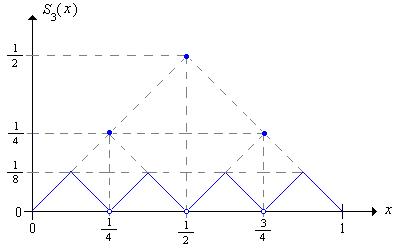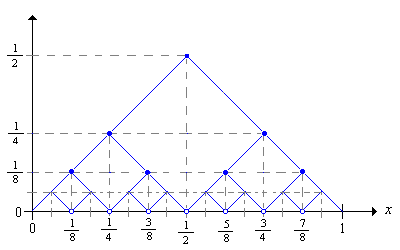]> Optimal Strategies

## 11. Optimal Strategies

Recall that the stopping rule for red and black is to continue playing until the gambler is ruined or her fortune reaches the target fortune $a$. Thus, the gambler's strategy is to decide how much to bet on each game before she must stop. Suppose that we have a class of strategies that correspond to certain valid fortunes and bets; $A$ will denote the set of fortunes and $B x$ will denote the set of valid bets for $x A$. For example, sometimes (as with timid play) we might want to restrict the fortunes to set of integers $0 1 a$; other times (as with bold play) we might want to use the interval $0 1$ as the fortune space. As for the bets, recall that the gambler cannot bet what she does not have and does not bet more than she needs in order to reach the target. Thus, a betting function $S$ must satisfy

$S x x a x , x A$

Moreover, we always restrict our strategies to those for which the stopping time is $N$ finite.

The success function of a strategy is the probability that the gambler reaches the target $a$ with that strategy, as a function of the initial fortune $x$. A strategy with success function $V$ is optimal if for any other strategy with success function $U$, we have $U x V x$ for $x A$

Show if there exists an optimal strategy, then the optimal success function is unique.

However, there may not exist an optimal strategy or there may be several optimal strategies. Moreover, the optimality question depends on the value of the game win probability $p$, in addition to the structure of fortunes and bets.

#### A Condition for Optimality

Our main theorem, and a sketch of its proof, are given in the following exercise:

A strategy $S$ with success function $V$ is optimal if

$p V x y q V x y V x , x A , y B x$
1. Consider the following strategy: if the initial fortune is $x A$, we pick $y A x$ and then bet $y$ on the first game; thereafter we follow strategy $S$. Condition on the outcome of the first game to show that the success function for this new strategy is $U x p V x y q V x y$.
2. Thus, the theorem can be restated as follows: If $S$ is optimal with respect to the class of strategies in part (a), then $S$ is optimal over all strategies.
3. Let $T$ be an arbitrary strategy with success function $U$. The random variable $V X n$ can be interpreted as the probability of winning if the gambler's strategy is replaced by strategy $S$ after time $n$.
4. Condition on the outcome of game $n$ to show that $V X n X 0 x p V X n 1 Y n q V X n 1 Y n X 0 x$.
5. Use (d) and the optimality condition to show $V X n X 0 x V X n 1 X 0 x$ for $n$ and $x A$.
6. Use (e) to show that $V X n X 0 x V x$ for $n$ and $x A$
7. Let $N$ denote the stopping time for strategy $T$. Let $n$ in (f) to show that $V X N X 0 x V x$ for $x A$.
8. Show that $V X N X 0 x U x , x A$.
9. Conclude that $U x V x$ for $x A$.

#### Favorable Trials with a Minimum Bet

Suppose now that $p 12$ so that the trials are favorable (or at least not unfair) to the gambler. Next, suppose that all bets must be multiples of a basic unit, which we might as well assume is \$1. Of course, real gambling houses have this restriction. Thus the set of valid fortunes is $A 0 1 a$ and the set of valid bets for $x A$ is $B x 0 1 x a x$. Our main result, and a sketch of its proof, are given in the following exercise:

Show that timid play is an optimal strategy.

1. Recall the success function $f$ for timid play.
2. Recall the optimality condition in Exercise 2.
3. Show first that optimality condition holds if $p 12$.
4. If $p 12$, show that the condition for optimality is equivalent to $p q p x y q q p x y q p x$
5. Show that the inequality in (d) is equivalent to $p q p y q y p y 1 q y 1 0$.
6. Show that the inequality in (e) holds for $p 12$.

In the red and black game set the target fortune to 16, the initial fortune to 8, and the game win probability to 0.45. Define the strategy of your choice and play 100 games. Compare your relative frequency of wins with the probability of winning with timid play.

#### Favorable Trials without a Minimum Bet

We will now assume that the house allows arbitrarily small bets and that $p 12$, so that the trials are strictly favorable. In this case it is natural to take the target as the monetary unit so that the set of fortunes is $A 0 1$, and the set of bets for $x A$ is $B x 0 x 1 x$. Our main result, and a sketch of the proof, are given in the following exercise. The results for timid play will play an important role in the analysis, so we will let $f j a$ denote the probability of reaching an integer target $a$, starting at the integer $j 0 a$, with unit bets.

Show that the optimal success function is $V x 1$ for $x 0 1$.

1. Fix a rational initial fortune $x k n 0 1$. Let $m$ be a positive integer and suppose that, starting at $x$, the gambler bets $1 m n$ on each game.
2. Show the strategy in (a) is equivalent to timid play with target fortune $m n$, and initial fortune $m k$,
3. The probability of reaching the target 1 under the strategy in (b) is $f m k m n$.
4. Show that $f m k m n 1$ as $m$.
5. From (d), show that $V x 1$ if $x 0 1$ is rational.
6. From (e) and the fact that $V$ is increasing to show that $V x 1$ for all $x 0 1$

#### Unfair Trials

We will now assume that $p 12$ so that the trials are unfair, or at least not favorable. As before, we will take the target fortune as the basic monetary unit and allow any valid fraction of this unit as a bet. Thus, the set of fortunes is $A 0 1$, and the set of bets for $x A$ is $B x 0 x 1 x$. The following exercise gives our main result and a sketch of the proof.

Show that bold play is optimal.

1. Let $F$ denote the success function for bold play, and let $D x y F x y 2 p F x q F y$
2. Show that the optimality condition is in Exercise 2 equivalent to $D x y 0$ for $0 x y 1$.
3. Use the continuity of $F$ to show that it suffices to prove the inequality in (b) when $x$ and $y$ are binary rationals.
4. Show that the inequality in (b) holds when $x$ and $y$ have rank 0: $x 0$, $y 0$; $x 0$, $y 1$; and $x 1$, $y 1$.
5. Suppose that the inequality in (b) holds when $x$ and $y$ have rank $m$ or less. For parts (f)-(k) below, suppose that $x$ and $y$ have rank $m 1$ or less.
6. Suppose that $x y 12$. Show that $D x y p D 2 x 2 y$.
7. Suppose that $12 x y$. Show that, $D x y q D 2 x 1 2 y 1$.
8. Suppose that $x x y 2 12 y$ and that $2 y 1 2 x$. Show that $D x y q p F 2 y 1 q D 2 y 1 2 x$
9. Suppose that $x x y 2 12 y$ and that $2 x 2 y 1$. Show that $D x y q p F 2 x q D 2 x 2 y 1$
10. Suppose that $x 12 x y 2 y$ and that $2 y 1 2 x$. Show that $D x y p q p 1 F 2 x p D 2 y 1 2 x$
11. Suppose that $x 12 x y 2 y$ and that $2 x 2 y 1$. Show that $D x y p q p 1 F 2 y 1 p D 2 x 2 y 1$
12. Use the induction hypothesis in (e) and parts (f)-(k) to finish the proof that bold play is optimal when the trials are unfair.

In the red and black game, set the target fortune to 16, the initial fortune to 8, and the game win probability to 0.45. Define the strategy of your choice and play 100 games. Compare your relative frequency of wins with the probability of winning with bold play.

#### Other Optimal Strategies in the Sub-Fair Case

Consider again the sub-fair case where $p 12$ so that the trials are not favorable to the gambler. We will show that bold play is not the only optimal strategy; amazingly, there are infinitely many optimal strategies. Recall first that the bold strategy has betting function

$S 1 x x 1 x x 0 x 12 1 x 12 x 1$Now consider the following strategy, which we will refer to as the second order bold strategy:

• With fortune $x 0 12$, play boldly with the object of reaching $12$ before falling to 0.
• With fortune $x 12 1$, play boldly with the object of reaching 1 without falling below $12$.
• With fortune $12$, bet $12$.

Show that the second order bold strategy has betting function $S 2$ given by

1. $S 2 x x$ if $x 0 14$
2. $S 2 x 12 x$ if $x 14 12$
3. $S 2 12 12$
4. $S 2 x x 12$ if $x 12 34$
5. $S 2 x 1 x$ if $x 34 1$Show that the second order bold strategy is optimal.

1. Let $F 2$ denote the success function associated with strategy $S 2$.
2. Suppose that the player starts with fortune $x 0 12$ under strategy $S 2$. Note that the player reaches the target 1 if and only if she reaches $12$. and then wins the final game.
3. In the setting of (b), consider the sequence of fortunes until the player reaches 0 or $12$. Argue that if we double the fortunes, then we have the fortune sequence under the ordinary bold strategy, starting at $2 x$ and terminating at either 0 or 1.
4. From parts (b) and (c) argue that $F 2 x p F 2 x$.
5. Suppose that the player starts with fortune $x 12 1$ under strategy $S 2$. Note that the player reaches the target 1 if and only if she reaches 1 without falling back to $12$ or falls back to $12$ and then wins the final game.
6. In the setting of (b), consider the sequence of fortunes until the player reaches $12$ or 1. Argue that if we double the fortunes and subtract 1, then we have the fortune sequence under the ordinary bold strategy, starting at $2 x 1$ and terminating at either 0 or 1.
7. Argue from (e) and (f) that $F 2 x F 2 x 1 1 F 2 x 1 p$.
8. Using (d) and (g) and the functional equation for ordinary bold play, conclude that $F 2 x F x$ for all $x 0 1$, and hence that .$S 2$ is optimal.

Once we understand how this construction is done, it's straightforward to define the third order bold strategy and show that it's optimal as well. The graph of the betting function is shown below:Explicitly give the third order betting function and show that the strategy is optimal.

More generally, we can define the $n$ order bold strategy and show that it is optimal as well.

Show that the sequence of bold strategies can be defined recursively from the basic bold strategy $S 1$ as follows:

1. $S n 1 x 12 S n 2 x$ if $x 0 12$
2. $S n 1 x 12 S n 2 x 1$ if $x 12 1$
3. $S n 1 12 12$

Even more generally, we can define an optimal strategy $T$ in the following way: for each $x 0 1$ select $n x$ and let $T x S n x x$. The graph below shows a few of the graphs of the bold strategies. For an optimal strategy $T$, we just need to select, for each $x$ a bet on one of the graphs.